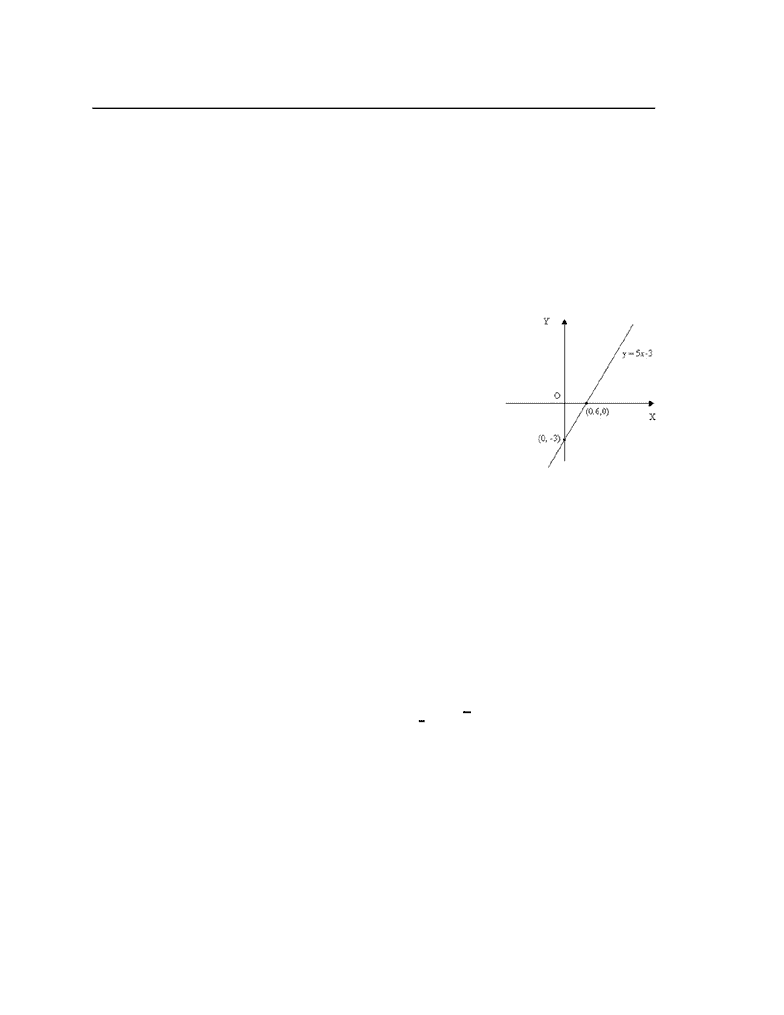# MATH 2A Lecture Notes - Lecture 2: Algebraic Function, Cube Root, Logarithm

121 views3 pages
School
Department
Course
ProfessorMATH 2A - Lecture 2 - Mathematical Models and DIfferent Types of Functions
A mathematical model is a mathematical description (often by means of function) or
(equations) of a real-world phenomenon.
Examples:
Speed of a falling object
Population
Concentration of a product in a chemical reaction
Life expectancy of a person at birth
Linear Models
The graph of the function is a line and it follows the
following format:
f(x|=mx+b
In the example on the right,
Slope, m = 3
Intercept b = -2
*image from MCA online
Polynomial function
A function f is called polynomial if:
(x)x x x .. x xf =ann+an−1 n−1 +an−2 n−2 +. +a22+a1+ao
are called polynomial coefficients,a... aa0 1, n
If , n is the degree of polynomial=an/ 0
Example:
(x)x xf = 2 6x4+5
23+ √2
Solution:
The polynomial is of degree 6
There are two famous types of polynomials
{f(x)=ax²+bx+c
{f(x)=ax³+bx²+cx+d
3-Power Function
Unlock document

This preview shows page 1 of the document.
Unlock all 3 pages and 3 million more documents.

## Document Summary

Math 2a - lecture 2 - mathematical models and different types of functions. A mathematical model is a mathematical description (often by means of function) or (equations) of a real-world phenomenon. Concentration of a product in a chemical reaction. Life expectancy of a person at birth. The graph of the function is a line and it follows the following format: A function f is called polynomial if: n 2 + . + a2 x n 1 + an 2 x n + an 1 x (x) x. = 2 6 x4 + 5 x. There are two famous types of polynomials. , where a is a constant is called a power function. Depending on the value of a we can have different shapes for power functions. = x (x) f: a = n and is a positive integer, a=1/n where n is a positive integer. Y = x y = 3 x root 3 or cube root: a=-1, y=1/x.

## Get access

\$8 USD/m\$10 USD/m
Billed \$96 USD annuallyHomework Help
Study Guides
Textbook Solutions
Class Notes
Textbook Notes
Booster Class